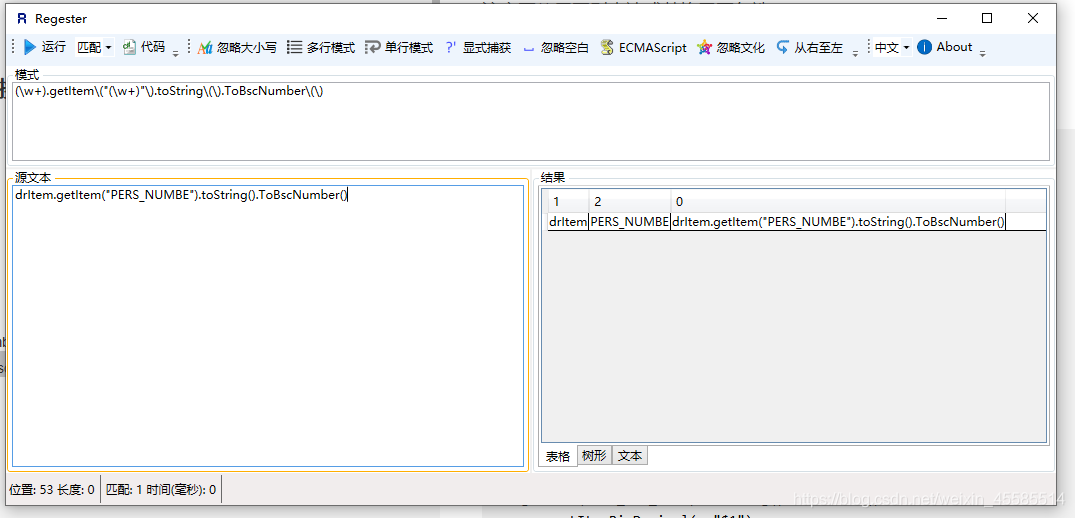﻿ idea 正则表达式搜索替换应用详解_正则表达式_脚本之家# idea 正则表达式搜索替换应用详解

idea 正则表达式搜索替换简单应用 利用正则表达式，idea可以进行快速大量替换

### 1.CTRL+R 快捷键调出下面当前页面替代操作栏### 2.利用正则表达式进行替换

```(\w+)get\(0\).getValue\("(\w+)"\)
dtCfwStget(0).getValue("CFW_CD")
\$1.getValue(0,"\$2")
dtCfwSt.getValue(0,"CFW_CD")

(\w+).getItem\("(\w+)"\).toString\(\).ToBscNumber\(\)
drItem.getItem("PERS_NUMBE").toString().ToBscNumber()
\$1.getItemBscNumber("\$2")
drItem.getItemBscNumber("PERS_NUMBE")

: (\w+).Rows\)
for (DataRow drItem : dtCardInfo.Rows)
: \$1.Rows())
for (DataRow drItem : dtCardInfo.Rows())

row.getItem\("(\w+)"\).toString\(\).ToDecimal\(\)
row.getItem("ACT_MTH_VAL").toString().ToDecimal()
row.getItemBigDecimal(	"\$1")
row.getItemBigDecimal(	"ACT_MTH_VAL")

\[0\]\["(\w+)"\]
matAssResRows["ASS_VAL"]
.get(0).getItem("\$1")
matAssResRows.get(0).getItem("ASS_VAL")``````drItem.getItem("PERS_NUMBE").toString().ToBscNumber()
drItaaa.getItem("PERS_bbb").toString().ToBscNumber()
drItemcccc.getItem("PERS_NUMBEccccc").toString().ToBscNumber()```

```drItem.getItemBscNumber("PERS_NUMBE")
drItaaa.getItemBscNumber("PERS_bbb")
drItemcccc.getItemBscNumber(PERS_NUMBEccccc")```

```// drItem.getItem("PERS_NUMBE").toString().ToBscNumber();
// drItem.getItem\("PERS_NUMBE"\).toString\(\).ToBscNumber\(\);```// X.getItem("X").toString().ToBscNumber();

// （/w+）.getItem\("(\w+)"\).toString\(\).ToBscNumber\(\);```

```这是我最终需要代码的一种
//drItem.getItemBscNumber("PERS_NUMBE")

// X.getItemBscNumber("X")

X.getItem("X").toString().ToBscNumber();

\$1.getItemBscNumber("\$2")```